# Final object

(diff) ← Older revision | Latest revision (diff) | Newer revision → (diff)

terminal object, of a category

A concept formalizing the categorical property of a one-point set. An objectin a category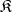is called final if for every objectinthe set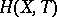consists of a single morphism. A final object is also called a right null object of. A left null or initial object of a category is defined in the dual way.

In the category of sets the final objects are just the one-point sets. In any category with null objects the final objects are the null objects. Other examples of final objects arise in various categories of diagrams, where the concept of a final object is essentially equivalent to that of the limit of a diagram. For example, suppose thatand let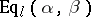be the category of left equalizers of the pair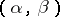; in other words, the objects ofare morphismsfor which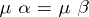, and morphisms inare morphisms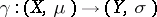for which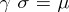. A final object inis a kernel of the pair of morphisms(cf. Kernel of a morphism in a category).

The setis, by definition, the set of morphisms. Note that any two final objects of a category are (canonically) isomorphic, and so are two initial objects.